# Electrostatics Force Discharge Potential Notes Class 12 Pdf Downl

chapter 1 ELECTROSTATICS

• Electrostatics is the study of charges at rest.
• The intrinsic property of fundamental particle of matter which give rise to electric force between objects is called charge.
• Charging a body can be done by friction, induction and conduction.
• Properties of charges:

o Like charges repel and unlike charges attract.
o Charges are additive in nature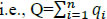o Charges are quantized. i.e., Q= ± ne [n=1,2,3,… & e=1.602 X10-19 C]
o Charge on a body is independent of velocity of the body.
o Charge is conserved.

• The sensitive device which is used to identify whether the body is charged or not is called electroscope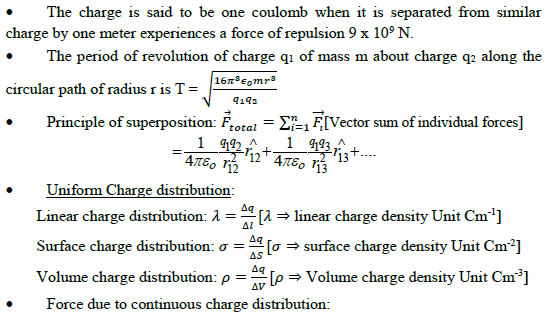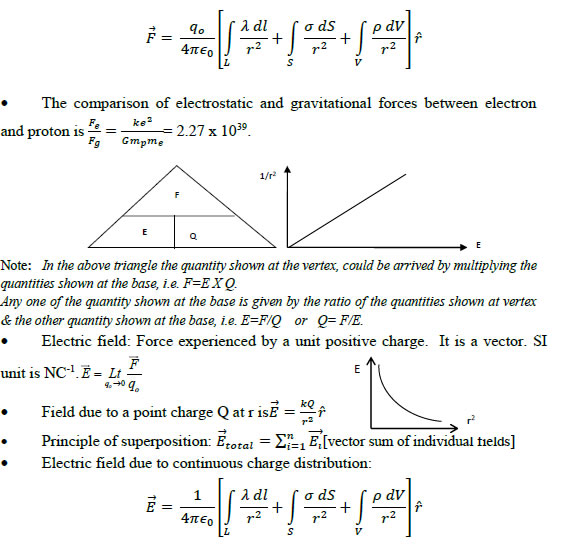• Dipole : Two equal and opposite charges separated by a small distance.
• Dipole moment: Product of magnitude of either charge and distance of separation between them. It is a vector. SI unit: Cm,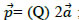direction of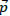is from negative charge to positive charge along the straight line joining both the charges.
• Dipole in a uniform electric field experiences no net translating force but experiences a torque.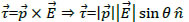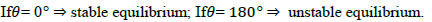• Electric field due to a short dipole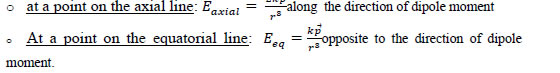##### ♦ Properties of electric field lines:

✓Arbitrarily starts from +ve charge and end at –ve charge
✓ Continuous, without any breaks, never form closed loops
✓Never intersect
✓Relative closeness of the field lines represents the magnitude of the fieldstrength.
✓For a set of two like charges – lateral pressure in between
✓For a set of two unlike charges – longitudinal contraction in between.

##### ♦ Area vector: The vector quantity representing the area of a surface whosemagnitude is equal to the magnitude of the area and direction is perpendicular to the surface.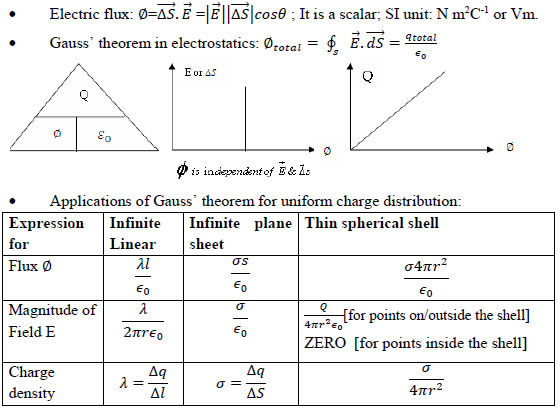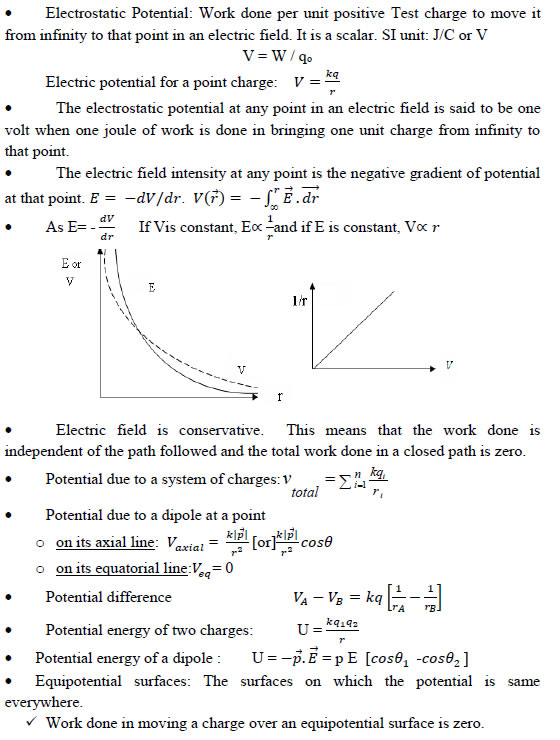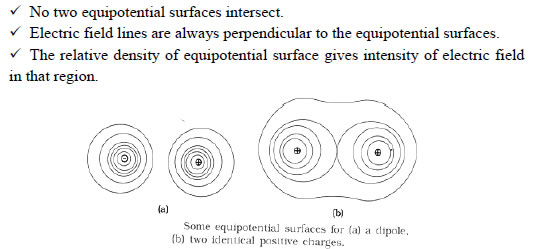##### • Electrostatics of conductors

(i) Inside a conductor Electrostatic field is zero
(ii) On the surface E is always Normal to the surface
(iii) No excess charge resides inside the conductor
(iv) Charge distribution on the surface is uniform if the surface is smooth
(v) Electric field is zero in the cavity of hollow conductor and potential remains constant which is equal to that on the surface.
• Capacitor: An arrangement of two conductors separated by a small distance without any electrical contact between them is called capacitor.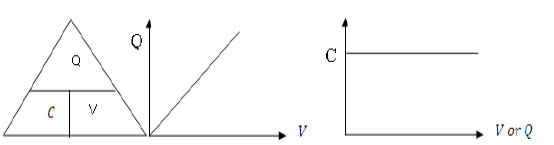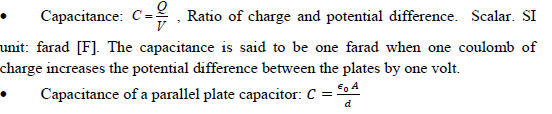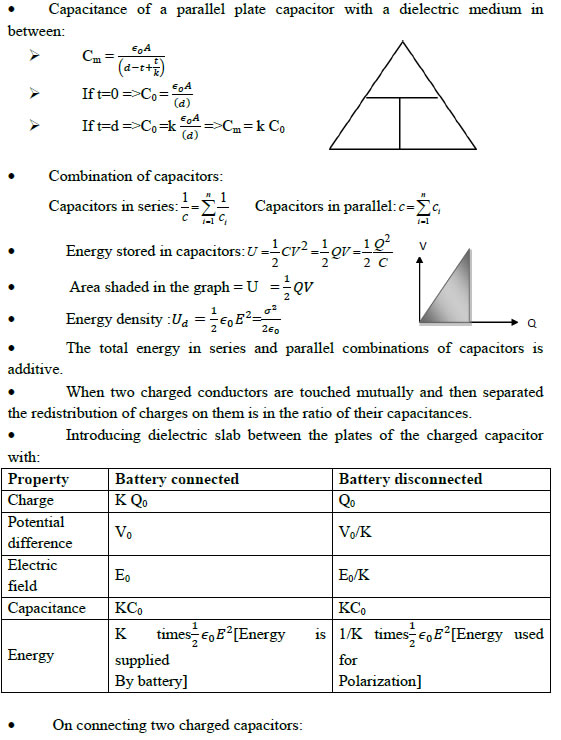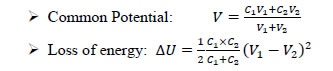• The dielectric is the substance which is essentially an insulator but behaves like a conductor in electrostatic situation.
• The dielectric having atom or molecules whose negative charge centre is not coinciding with positive charge centre is called polar dielectric. They have permanent dipole moments in the order of 10-30 Cm.
• The dielectric having atom or molecules whose negative charge centre is coinciding with positive charge centre is called non-polar dielectric.
• The dipole moment developed in non-polar dielectric due to external electric field is called induced dipole moment.
• The induced dipole moment per unit volume is called Polarisation Vector. The direction of polarisation vector is same as that of external electric filed.
• The ratio of electrostatic force in free space to that in medium OR the ratio of electrostatic field in free space to that in medium OR the ratio of absolute permittivity of medium to that of free space is called relative permittivity or dielecric constant of the medium.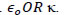• The ratio of polarisation to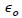times the electric field intensity is called electric susceptibility.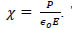he dielectrics with constant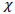are called linear dielectrics.
• The maximum external electric field the dielectric can withstand without dielectric beakdown is called dielectric strength. SI unit Vm-1
• The capacitance of a spherical conductor of radius R is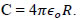### NCERT Books Free Pdf Download for Class 5, 6, 7, 8, 9, 10 , 11, 12 Hindi and English Medium

 Mathematics Biology Psychology Chemistry English Economics Sociology Hindi Business Studies Geography Science Political Science Statistics Physics Accountancy